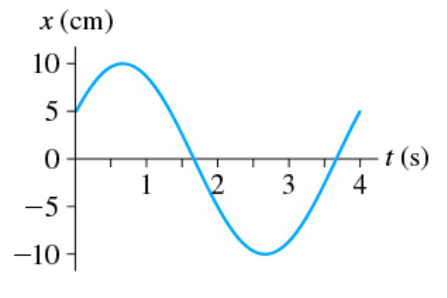# Problem: (Figure 1) is the position-versus-time graph of a particle in simple harmonic motion.(a) What is the phase constant?(b) What is the velocity at t=0s?(c) What is vmax?

###### FREE Expert Solution
88% (158 ratings)
###### FREE Expert Solution

a.)

Angular frequency,

$\overline{)\begin{array}{rcl}{\mathbf{\omega }}& {\mathbf{=}}& \frac{\mathbf{2}\mathbf{\pi }}{\mathbf{T}}\end{array}}$

T = 4 s

ω = 2π/4 = π/2 rad/s

For Simple harmonic motion,

Solving for Φ,

A = 10 cm = 0.1 m

x(t) = 5 cm = 0.05

t = 0 s

$\begin{array}{rcl}\mathbf{\varphi }& \mathbf{=}& {\mathbf{cos}}^{\mathbf{-}\mathbf{1}}\mathbf{\left(}\frac{{\mathbf{x}}{\mathbf{\left(}}{\mathbf{t}}{\mathbf{\right)}}}{\mathbf{A}}\mathbf{\right)}\mathbf{-}{\mathbf{\omega t}}\\ & \mathbf{=}& {\mathbf{cos}}^{\mathbf{-}\mathbf{1}}\mathbf{\left(}\frac{\mathbf{0}\mathbf{.}\mathbf{05}}{\mathbf{0}\mathbf{.}\mathbf{1}}\mathbf{\right)}\mathbf{-}{\mathbf{\omega }}{\mathbf{·}}{\mathbf{0}}\end{array}$

88% (158 ratings)###### Problem Details

(Figure 1) is the position-versus-time graph of a particle in simple harmonic motion.

(a) What is the phase constant?
(b) What is the velocity at t=0s?
(c) What is vmax?